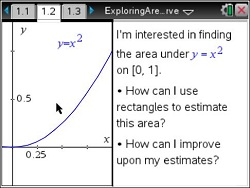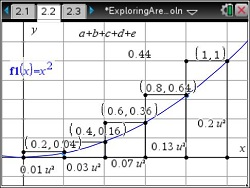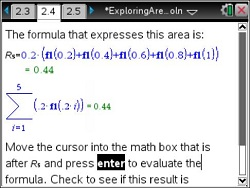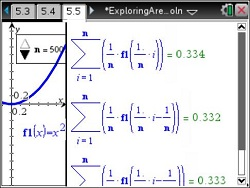# Activities

••• ##### Subject Area

• Math: Calculus: Antiderivatives and Slope Fields

• ##### Author9-12

45 Minutes

• ##### Device
•TI-Nspire™ CX/CX II
•TI-Nspire™ CX CAS/CX II CAS
• TI-Nspire™
• TI-Nspire™ CAS
• ##### Software

TI-Nspire™
TI-Nspire™ CAS

3.2

## Exploring the Area Under a Curve

#### Activity Overview

Students explore Riemann sums to find the approximate area under the graph of a parabola on the interval [0, 1].

#### Key Steps

•Students focus on two questions that define the activity:

How can you use rectangles to approximate the area under the curve y=y 2 and above the x-axis.

Is there a way to use rectangles to find the exact area under the curve?

•Students make use of five right-endpoint rectangles to find an approximation of the area under the curve.

Students will be introduced to the Riemann sum. They explore the Riemann sum with right-endpoints.

•Next, students use five left-endpoint rectangles to find an approximation of the are under the curve.

•Finally, students summarize their findings. They will notice that the concavity of a function and whether it is increasing or decreasing will determine which estimates are overestimates verses underestimates.